## Latest:

latest

### Logic gate

A logic gate is an idealized or physical device, which implements a Boolean function. Different types of logic gates are used in different cases.

That is, it performs a logical operation on one or more binary inputs and produces a single binary output. It is playing a very important role in electronics.

It is a basic element. Using a lot of gates many big devices are created.

### Types of logic gate

1. Basic logic gates: AND, OR, NOT
2. Universal gates: NAND, NOR
3. Exclusive gates: X-OR, X-NOR
4. Complex/Compound gates: AOI and OAI complex gates
5. Buffer gate

### Basic logic gate

Here we are discussing basic logic gates. They are AND, OR, NOT.

#### AND gate

This circuit has two or more inputs and it gives produces only a single output which is the logical AND operation of all inputs. We represent this logical operation by . sign.

If A and B are two inputs of a logical AND gate then output X will be

X = A.B

The truth table for the AND gate of two inputs is given below.

 A B X 0 0 0 0 1 0 1 0 0 1 1 1

Here, if both inputs are 1 then the output is 1. Otherwise, the output is 0.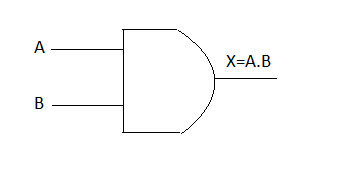AND gate

AND gate is used for making a security alarm system and also in different machine security. By 0 and 1 any unique condition can be generated. That helps for securing the systems.

#### OR gate

This circuit has two or more inputs and it gives produces only a single output which is the logical OR is the operation of all inputs. We represent this logical operation by + sign.

If A and B are two inputs of a logical OR gate then output X will be

X = A+B

The truth table for the OR gate of two inputs is given below.

 A B X 0 0 0 0 1 1 1 0 1 1 1 1

Here, if one of the inputs is 1 then we will get 1 as output.

#### NOT gate

This logical circuit has only a single input and we also get only a single output from this. The output is the inversion of the given input. This gate is also called an inverter. We represent this logical operation by the "¯" sign. It complements the input.

If A is the input of a logical NOT gate then output X will be

X= Ā

The truth table for NOT gate is given below.

 A X =Ā 0 1

If the input is 0 then output is 1 and if the input is 1 then it will give 0 as output.

## Universal gates are that type of gate by using that we can implement any type of Boolean functions.

A digital circuit is designed using different logic gates. But only using a single type of gate we can make the digital circuit more simply.

Two types of Universal gates are
1. NAND
2. NOR

## We can implement any type of Boolean functions by using NAND and NOR gates. Because of this, these two gates are called the universal gates.

### This gate takes more than a single input and gives a single output which is the inversion of logical AND operation of those inputs.

If A and B are two inputs of a logical NAND gate then output X will be

X= A͞.B

The truth table for a NAND  gate of two inputs is given below.

 A B X 0 0 1 0 1 1 1 0 1 1 1 0

Here, if both inputs are 1 then the output is 0. Otherwise, the output is 1.

#### NOR gate

This gate takes more than a single input and gives a single output which is the inversion of logical OR  operation of those inputs.

If A and B are two inputs of a logical NOR gate then output X will be

A truth table for the NOR gate of two inputs is given below.

 A B X 0 0 1 0 1 0 1 0 0 1 1 0

### The Universality of NAND gate

Other logical gates are preventable by using the NAND gate.

#### AND gate by using NAND gate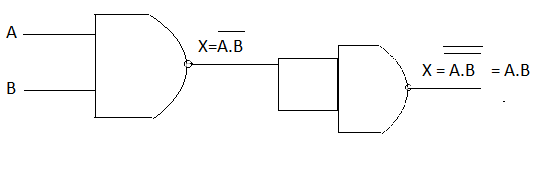AND gate by using NAND gate

### For representing the OR gate by using the NAND gate we have used three NAND gates.

#### NOT gate by using NAND gate

For representing NOT gate by using the NAND gate we have used a single NAND gate.

#### NOR gate by using NAND gate

For representing the NOR gate by using the NAND gate we have used four NAND gates.

### The universality of NOR gate

Other logical gates can be designed by using the NOR gate.

#### AND gate by using NOR gate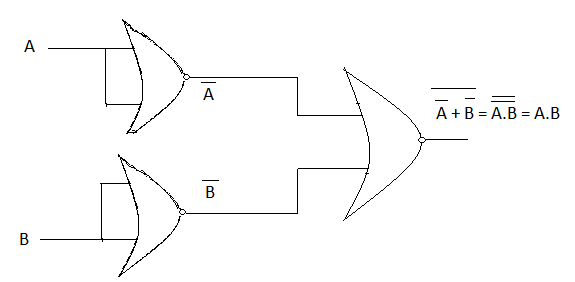AND gate by using NOR gate

For representing AND gate by using the NOR gate we have used three NOR gates.

#### OR gate by using NOR gateOR gate by using NOR gate

### For representing the OR gate by using the NOR gate we have used two NOR gates.

#### NOT gate by using NOR gate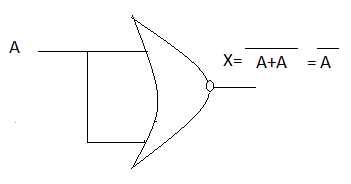NOT gate by using NOR gate

### For representing the X-OR gate by using the NOR gate we have used 5  NOR gates.

For representing the X-NOR gate by using the NOR gate we have used 5 NOR gates.

### Exclusive gates

Two exclusive gates are:
1. X-OR(Exclusive OR) gate
2. X-NOR(Exclusive NOR) gate

#### X-OR gate

This circuit has two or more inputs and it gives produces only a single output which is the logical X-OR operation of all inputs. The output will be high when both inputs are different and will be low when inputs are the same.

We represent this logical operation by " ⊕ " sign.

If A and B are two inputs of a logical X-OR gate then output X will be-

X = A⊕B
Here,
X = ĀB+AB̄

The truth table for the X-OR gate of two inputs is given below.

 A B X 0 0 0 0 1 1 1 0 1 1 1 0

Here, if both inputs are 1 or both are 0 then the output is 0. Otherwise, the output is 1.

Fig: X-OR gate

#### X-NOR gate

This circuit has two or more inputs and it gives produces only a single output which is the logical X-NOR operation of all inputs. The output will be high when both inputs are the same and will be low when inputs are different.
If A and B are two inputs of a logical X-NOR gate then output X will be

Here,
X= AB+ĀB̄

A truth table for the X-NOR gate of two inputs is given below.

 A B X 0 0 1 0 1 0 1 0 0 1 1 1

#### *Buffer gate

Buffer gate
In the amplifier buffer gate is used.  Buffer gate does an interesting task. It amplifies the signal. For increasing propagation delay of signal buffer gate is used.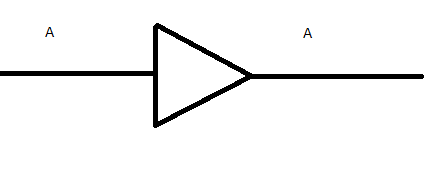Buffer gate

The truth table of the buffer gate

Here we will get the output as like input.

 Input Output 0 0 1 1

So, here we have discussed-

1. Logic gates
2. Types of logic gate
3. Basic logic gates: AND, OR, NOT
4. Universal gates: NAND gate, NOR gate, Universality of the universal gates.
5. Exclusive gates: X-OR, X-NOR